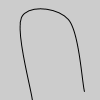Name

### curveVertex()

Examples```noFill()
beginShape()
curveVertex(84, 91)
curveVertex(84, 91)
curveVertex(68, 19)
curveVertex(21, 17)
curveVertex(32, 100)
curveVertex(32, 100)
endShape()
```
Description Specifies vertex coordinates for curves. This function may only be used between beginShape() and endShape() and only when there is no MODE parameter specified to beginShape(). The first and last points in a series of curveVertex() lines will be used to guide the beginning and end of a the curve. A minimum of four points is required to draw a tiny curve between the second and third points. Adding a fifth point with curveVertex() will draw the curve between the second, third, and fourth points. The curveVertex() function is an implementation of Catmull-Rom splines. Using the 3D version requires rendering with P3D (see the Environment reference for more information).
Syntax
```curveVertex(x, y)
curveVertex(x, y, z)
```
Parameters
x float: the x-coordinate of the vertex float: the y-coordinate of the vertex float: the z-coordinate of the vertex
Related curve()
beginShape()
endShape()
vertex()
bezier()